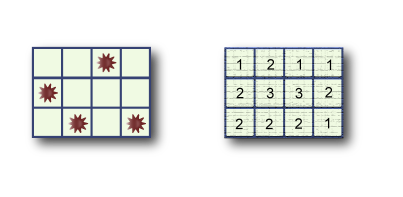시간 제한 메모리 제한 제출 정답 맞은 사람 정답 비율
5 초 512 MB 8 6 5 71.429%

## 문제

MineLayer is a MineSweeper-like puzzle game played on an R by C grid. Each square in the grid either has one mine or no mines at all. A MineLayer puzzle consists of a grid of numbers, each of which indicates the total number of mines in all adjacent squares and in the square underneath. The numbers will thus range from zero to nine.

The objective of MineLayer is to figure out a layout of the mines in the grid that matches the given clues.

Below is a typical 3 by 4 grid. The original layout is on the left, and the puzzle on the right.Since there may be many solutions, your task is to write a program that outputs the maximum possible number of mines in the middle row. The number of rows will always be odd, and there will always be at least one solution to the puzzle.

## 입력

The first line of input gives the number of cases, NN test cases follow.

The first line of each case contains two space-separated numbers: R, the number of rows, and C, the number of columns. R is always an odd integer. Each of the next R lines contains C space-separated numbers that denote the clues of that row.

Limits

• 1 ≤ N ≤ 50.
• Each puzzle is guaranteed to have at least one solution.
• R = 3 or R = 5.
• 3 ≤ C ≤ 5.

## 출력

For each test case, output one line containing "Case #X: Y", where X is the 1-based case number, and Y is the maximum possible number of mines in the middle row of a grid that satisfies the given constraints.

## 예제 입력 1

2
3 3
2 2 1
3 4 3
2 3 2
3 4
1 2 1 1
2 3 3 2
2 2 2 1


## 예제 출력 1

Case #1: 1
Case #2: 1


## 채점 및 기타 정보

• 예제는 채점하지 않는다.